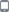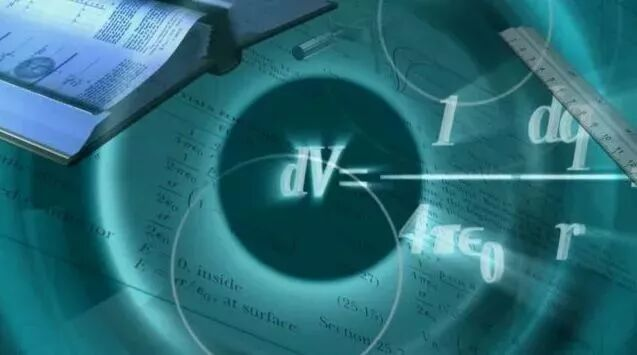• 当前城市【嘉兴
•  |
•|
|
|
|
|

|
|
|小学数学单位换算公式大全及专项训练
 嘉兴课外培训，嘉兴草屋网   2019-02-22 12:15:02   作者：亿欧   来源：   文字：【大】【中】【小】

01

1吨=1000千克   1吨=1000 000克

1千克=1000克   500克=1斤

1千克=1公斤   1公斤=2斤

02

1元=10角

1角=10分

1元=100分

03

1世纪=100年       1年=12个月

1日=24小时    1时=60分

1分=60秒     1时=3600秒04

1千米（km）=1000米(m)，1米(m)=10分米(dm)。

1千米（km）=1000米(m)=10000分米（dm）=1000 00厘米（cm）=1000 000毫米（mm）
1米(m)=10分米(dm)=100厘米（cm）=1000毫米（mm）
1分米(dm)=10厘米(cm)=100毫米（mm）
1厘米(cm)=10毫米(mm）

05

1平方千米=100公顷

1公顷=10000平方米

1平方米=100平方分米

1平方分米=100平方厘米

1平方厘米=100平方毫米

06

1立方米=1000立方分米；1立方分米=1000立方厘米

1升=1000毫升；1升=1立方分米1毫升=1立方厘米1立方米=1000立方分米

1立方分米=1000立方厘米；1立方分米=1升； 1立方厘米=1毫升；1立方米=1000升

单位换算练习题（一）

0.15千克=（   ）克         3.001吨=（  ）吨（  ）千克
3.7平方分米=（   ）平方毫米    5.80元=（  ）元（  ）角
(    )分米=1.5米         (    )吨(    )千克=4.08吨
510米=(    )千米         5米16厘米=(    )米
5千克700克=(    )千克    0.95米=(    )厘米
4700米=(    )千米    3650克=(    )千克    40.06吨=(    )千克

1.4平方米=(    )分米   360平方米=(    )公顷

7.05米＝（   ）米（   ）厘米    5.45千克＝（  ）千克（  ）克
3千米50米＝（    ）千米       （   ）时＝30分
3千克500克＝（   ）千克     （   ）时＝2时45分

2.78吨＝（   ）吨（   ）千克     0.25时＝（   ）分
504厘米＝（    ）米      4.2米＝（    ）米（    ）厘米
10米7分米＝（    ）米     0.06平方千米＝（    ）公顷
9千克750克＝（   ）千克     8.04吨＝（  ）吨（  ）千克

◇ 推荐资讯
◇ 资讯搜索
 类别： 请选择资讯类别 嘉兴教育招聘会培训动态相关知识培训资讯站内通告小学生阅读人教版北师大版苏教版相关资源各类试卷语文资源英语资源 关键：
◇ 新增企业

Copyright © 2002 - 2020 jxcww.net 备案号：浙ICP备15010677号-1